## Example Questions

← Previous 1

### Example Question #1 : How To Multiply Exponents

(b * b* b7)1/2/(b3 * bx) = b5

If b is not negative then x = ?

–1

1

–2

7

–2

Explanation:

Simplifying the equation gives b6/(b3+x) = b5.

In order to satisfy this case, x must be equal to –2.

### Example Question #2 : How To Multiply Exponents

If〖7/8〗n= √(〖7/8〗5),then what is the value of n?

2/5

√5

5/2

1/5

25

5/2

Explanation:

7/8 is being raised to the 5th power and to the 1/2 power at the same time. We multiply these to find n.

### Example Question #3 : How To Multiply Exponents

Quantity A:

(0.5)3(0.5)3

Quantity B:

(0.5)7

Quantity A is greater.

The relationship cannot be determined from the information given.

The two quantities are equal.

Quantity B is greater.

Quantity A is greater.

Explanation:

When we have two identical numbers, each raised to an exponent, and multiplied together, we add the exponents together:

xaxb = xa+b

This means that (0.5)3(0.5)3 = (0.5)3+3 = (0.5)6

Because 0.5 is between 0 and 1, we know that when it is multipled by itself, it decreases in value. Example: 0.5 * 0.5 = 0.25. 0.5 * 0.5 * 0.5 = 0.125. Etc.

Thus, (0.5)6 > (0.5)7

### Example Question #21 : Exponential Operations

For the quantities below, x<y and x and y are both integers.

Please elect the answer that describes the relationship between the two quantities below:

Quantity A

x5y3

Quantity B

x4y4

The quantities are equal.

The relationship cannot be determined from the information provided.

Quantity B is greater.

Quantity A is greater.

The relationship cannot be determined from the information provided.

Explanation:

Answer: The relationship cannot be determined from the information provided.

Explanation: The best thing to do here is to notice that quantity A is composed of two complex terms with odd exponents. Odd powers result in negative results when their base is negative. Thus quantity A will be negative when either x or y (but not both) is negative. Otherwise, quantity A will be positive. Quantity B, however, has two even exponents, meaning that it will always be positive. Thus, sometimes Quantity A will be greater and sometimes Quantity B will be greater. Thus the answer is that the relationship cannot be determined.

### Example Question #1561 : Gre Quantitative Reasoning

Simplify: (x3 * 2x4 * 5y + 4y2 + 3y2)/y

10x7y + 7y2

10x7 + 7y3

10x7 + 7y

10x11 + 7y3

10x7 + 7y

Explanation:

Let's do each of these separately:

x3 * 2x4 * 5y = 2 * 5 * x* x* y = 10 * x7 * y = 10x7y

4y2 + 3y2 = 7y2

Now, rewrite what we have so far:

(10x7y + 7y2)/y

There are several options for reducing this.  Remember that when we divide, we can "distribute" the denominator through to each member.  That means we can rewrite this as:

(10x7y)/y + (7y2)/y

Subtract the y exponents values in each term to get:

10x7 + 7y

### Example Question #1 : How To Multiply Exponents

Quantitative Comparison

Quantity A: x3/3

Quantity B: (x/3)3

Quantity B is greater.

Quantity A is greater.

The relationship cannot be determined from the information given.

The two quantities are equal.

The relationship cannot be determined from the information given.

Explanation:

First let's look at Quantity B:

(x/3)3 = x3/27. Now both columns have an xso we can cancel it from both terms. Therefore we're now comparing 1/3 in Quantity A to 1/27 in Quantity B.  1/3 is the larger fraction so Quantity A is greater.

However, if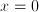, then the two quantities would both equal 0.  Thus, since the two quantities can have different relationships based on the value of, we cannot determine the relationship from the information given.

### Example Question #7 : How To Multiply Exponents

Quantitative Comparison: Compare Quantity A and Quantity B, using additional information centered above the two quantities if such information is given.

Quantity A          Quantity B

(23 )2                       (22 )3

Quantity A is greater.

Quantity B is greater.

The relationship cannot be determined from the information given.

The two quantities are equal.

The two quantities are equal.

Explanation:

The two quantites are equal. To take the exponent of an exponent, the two exponents should be multiplied.

(2)or 23*2 = 64

(2)or 22*3 = 64

Both quantities equal 64, so the two quantities are equal.

### Example Question #21 : Exponential Operations

Compare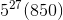and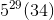.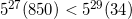The answer cannot be determined from the information given.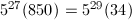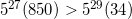Explanation:

To compare these expressions more easily, we'll change the first expression to have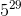in front. We'll do this by factoring out 25 (that is,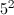) from 850, then using the fact that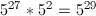.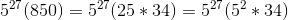When we combine like terms, we can see that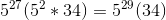. The two terms are therefore both equal to the same value.

### Example Question #9 : How To Multiply Exponents

Which of the following is equal to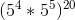?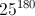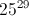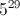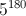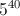Explanation: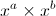is always equal to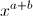; therefore, 5 raised to 4 times 5 raised to 5 must equal 5 raised to 9.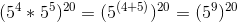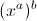is always equal to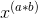. Therefore, 5 raised to 9, raised to 20 must equal 5 raised to 180.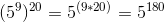### Example Question #10 : How To Multiply Exponents

Which of the following is equal to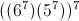?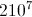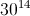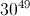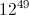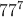Explanation:

First, multiply inside the parentheses: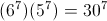.

Then raise to the 7th power: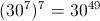.

← Previous 1

Tired of practice problems?

Try live online GRE prep today.